# Implementing an Unordered List

In order to implement an unordered list, we will construct what is commonly known as a linked list. Recall that we need to be sure that we can maintain the relative positioning of the items. However, there is no requirement that we maintain that positioning in contiguous memory. For example, consider the collection of items shown below. It appears that these values have been placed randomly.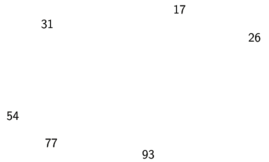Items not constrained in their physical placement

If we can maintain some explicit information in each item, namely the location of the next item, then the relative position of each item can be expressed by simply following the link from one item to the next:

It is important to note that the location of the first item of the list must be explicitly specified. Once we know where the first item is, the first item can tell us where the second is, and so on. The external reference is often referred to as the head of the list. Similarly, the last item needs to know that there is no next item.

## The Node Class

The basic building block for the linked list implementation is the node. Each node object must hold at least two pieces of information. First, the node must contain the list item itself. We will call this the data field of the node. In addition, each node must hold a reference to the next node. Here we provide one simple Python implementation:

class Node(object):
def __init__(self, value):
self.value = value
self.next = None


To construct a node, you need to supply the initial data value for the node. Evaluating the assignment statement below will yield a node object containing the value passed:

>>> temp = Node(93)
>>> temp.value
93


The special Python reference value None will play an important role in the Node class and later in the linked list itself. A reference to None will denote the fact that there is no next node. Note in the constructor that a node is initially created with next set to None. Since this is sometimes referred to as “grounding the node,” we will use the standard ground symbol to denote a reference that is referring to None. It is always a good idea to explicitly assign None to your initial next reference values.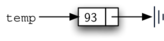A typical representation for a node

## The Unordered List Class

As we suggested above, the unordered list will be built from a collection of nodes, each linked to the next by explicit references. As long as we know where to find the first node (containing the first item), each item after that can be found by successively following the next links. With this in mind, the UnorderedList class must maintain a reference to the first node. Below we show the constructor. Note that each list object will maintain a single reference to the head of the list.

class UnorderedList(object):

def __init__(self):



Initially when we construct a list, there are no items. The assignment statement

>>> mylist = UnorderedList()



creates this linked list representation:

As we discussed in the Node class, the special reference None will again be used to state that the head of the list does not refer to anything. Eventually, the example list given earlier will be represented by this linked list:

The head of the list refers to the first node which contains the first item of the list. In turn, that node holds a reference to the next node (the next item) and so on. It is important to note that the list class itself does not contain any node objects. Instead it contains a single reference to only the first node in the linked structure.

The is_empty method, shown below, simply checks to see if the head of the list is a reference to None. The result of the boolean expression self.head is None will only be true if there are no nodes in the linked list. Since a new list is empty, the constructor and the check for empty must be consistent with one another. This shows the advantage to using the reference None to denote the “end” of the linked structure. In Python, None can be compared to any reference. Two references are equal if they both refer to the same object. We will use this often in our remaining methods.

    def is_empty(self):
return self.head is None


So, how do we get items into our list? We need to implement the add method. However, before we can do that, we need to address the important question of where in the linked list to place the new item. Since this list is unordered, the specific location of the new item with respect to the other items already in the list is not important. The new item can go anywhere. With that in mind, it makes sense to place the new item in the easiest location possible.

Recall that the linked list structure provides us with only one entry point, the head of the list. All of the other nodes can only be reached by accessing the first node and then following next links. This means that the easiest place to add the new node is right at the head, or beginning, of the list. In other words, we will make the new item the first item of the list and the existing items will need to be linked to this new first item so that they follow.

The linked list shown above was built by calling the add method a number of times.

>>> mylist.add(31)


Note that since 31 is the first item added to the list, it will eventually be the last node on the linked list as every other item is added ahead of it. Also, since 54 is the last item added, it will become the data value in the first node of the linked list.

The add method is shown below. Each item of the list must reside in a node object. We create a new node within the method and place the item as its value. Then we complete the process by linking the new node into the existing structure.

    def add(self, item):
temp = Node(item)


This requires two steps as shown below. Step 1 (line 3) changes the next reference of the new node to refer to the old first node of the list. Now that the rest of the list has been properly attached to the new node, we can modify the head of the list to refer to the new node.

The order of the two steps described above is very important. What happens if the order of the steps is reversed? If the modification of the head of the list happens first, the result can be seen below. Since the head was the only external reference to the list nodes, all of the original nodes are lost and can no longer be accessed.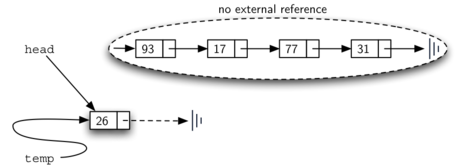Result of reversing the order of the two steps

The next methods that we will implement–size, search, and remove–are all based on a technique known as linked list traversal. Traversal refers to the process of systematically visiting each node. To do this we use an external reference that starts at the first node in the list. As we visit each node, we move the reference to the next node by “traversing” the next reference.

To implement the size method, we need to traverse the linked list and keep a count of the number of nodes that occurred. Below we provide the Python code for counting the number of nodes in the list. The external reference is called current and is initialized to the head of the list in line 2. At the start of the process we have not seen any nodes so the count is set to $0$. Lines 4–6 actually implement the traversal. As long as the current reference has not seen the end of the list (None), we move current along to the next node via the assignment statement in line 6. Every time current moves to a new node, we add $1$ to count. Finally, count gets returned after the iteration stops.

    def size(self):
count = 0
while current is not None:
count = count + 1
current = current.next

return count


Searching for a value in a linked list implementation of an unordered list also uses the traversal technique. As we visit each node in the linked list we will ask whether the data stored there matches the item we are looking for. In this case, however, we may not have to traverse all the way to the end of the list. In fact, if we do get to the end of the list, that means that the item we are looking for must not be present. Also, if we do find the item, there is no need to continue.

Here is a possible implementation of search:

    def search(self, item):

while current is not None:
if current.value == item:
return True
current = current.next

return False


The remove method requires two logical steps. First, we need to traverse the list looking for the item we want to remove. Once we find the item (recall that we assume it is present), we must remove it. The first step is very similar to search. Starting with an external reference set to the head of the list, we traverse the links until we discover the item we are looking for. Since we assume that item is present, we know that the iteration will stop before current gets to None.

Once we have found the node to be removed, how do we remove it? One possibility would be to replace the value of the item with some marker that suggests that the item is no longer present. The problem with this approach is the number of nodes will no longer match the number of items. It would be much better to remove the item by removing the entire node.

In order to remove the node containing the item, we need to modify the link in the previous node so that it refers to the node that comes after current. Unfortunately, there is no way to go backward in the linked list. Since current refers to the node ahead of the node where we would like to make the change, it is too late to make the necessary modification.

The solution to this dilemma is to use two external references as we traverse down the linked list. current will behave just as it did before, marking the current location of the traverse. The new reference, which we will call previous, will always travel one node behind current. That way, when current stops at the node to be removed, previous will be referring to the proper place in the linked list for the modification.

Here is an implementation of a complete remove method:

    def remove(self, item):
previous = None

while True:
if current.value == item:
break
previous, current = current, current.next

if previous is None:
else:
previous.next = current.next


First we assign current and previous to the head of the list and None respectively. Then, on each iteration of our while loop, we break if current represents the node we wish to remove, and if not we update previous and current to current and current.next respectively. Again, the order of these two statements is crucial. previous must first be moved one node ahead to the location of current. At that point, current can be moved.

Here we illustrate the movement of previous and current as they progress down the list looking for the node containing the value 17: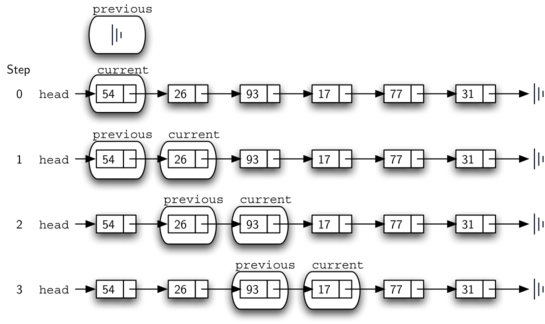"previous" and "current" move down the list

Once the searching step of the remove has been completed, we need to remove the node from the linked list. If previous is None, we know that current is in fact the head of the list, so we remove that node by updating the head of the list to the subsequent node, thereby losing the reference to the original head node:

In all other cases, we know that both previous and current are nodes in the list, so we can remove current by setting the next attribute of previous to the node after current in the list: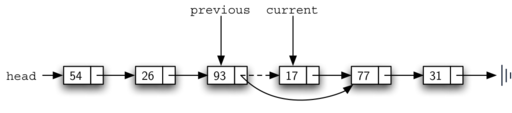Removing an item from the middle of the list

The remaining methods append, insert, index, and pop are left as exercises. Remember that each of these must take into account whether the change is taking place at the head of the list or someplace else. Also, insert, index, and pop require that we name the positions of the list. We will assume that position names are integers starting with 0.

# Practical Algorithms and Data Structures

Introduction

## Lists

1. Introduction to Lists
2. Implementing an Unordered List
3. Implementing an Ordered List